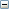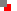﻿ NumbersEven(N:number):boolean;
 Function EVEN function returns TRUE if number N is not odd. See also ODD function, below.Odd(N:number):boolean;
 Function ODD function returns TRUE if number N is odd. See also EVEN function, above.Inc(var X [ ; N: number] );
 Procedure X is a number-type variable. N is an optional number-type expression. If N is specified then X increments by N; that is, INC(X, N) corresponds to the statement X := X + N. If N is not specified then X increments by 1; that is, INC(X) corresponds to the statement X := X + 1. Note that INC generates optimized code and is especially useful in tight loops. See also DEC procedure, below.Dec(var X[ ; N: number] );
 Procedure X is a number-type variable. N is a number-type expression. If N is specified then X decrements by N; that is, DEC(X, N) corresponds to the statement X := X - N. If N is not specified then X decrements by 1; that is, DEC(X) corresponds to the statement X := X - 1. Note that DEC generates optimized code and is especially useful in tight loops. See also INC procedure, above.Min(A,B: number): number;

Function

MIN returns the lower of the two values A and B; negative values are smaller than zero. Thus:

 When MIN returns Example A B MIN A>B B 2 1 1 AMax(A,B: number): number;

Function

MAX returns the higher of the two values A and B; negative values are smaller than zero. Thus:

 When MAX returns Example A B MAX A>B A 2 1 2 ARandom [Range: number]:number;IntToStr(N:number):string;
 Function INTTOSTRING converts number N to string representation.Rotate example, Column example See also STRINGTOINTDEF and STRTOFLOATDEF functions.